• python遍历一个矩阵
千次阅读
2021-03-18 10:32:14
matrix =[[1,2], [3,4]]
for i,element in enumerate(matrix):
print(i, element)


output:
(0,0) 1
(0,1) 2
(1,0) 3
(1,1) 4
遍历绝阵，这个是按照矩阵一行行进行遍历，输出矩阵的索引和元素

更多相关内容
• 关注微信公众号“酸痛鱼”，获得更多最新最全...0问题描述 实现一个函数，该函数接收一个n×n二维矩阵matrix，将该矩阵顺时针旋转90度。要求直接对参数matrix进行修改，函数不返回任务东西。例如：给定 matrix = [ ...

关注微信公众号“酸痛鱼”，获得更多最新最全的文章。

本文中所涉及的代码，在未特殊声明的情况下，都是基于Python3程序设计语言编写的。

建议您在PC浏览器中阅读本文，以获得更好的阅读体验。

0

问题描述

实现一个函数，该函数接收一个n×n二维矩阵matrix，将该矩阵顺时针旋转90度。要求直接对参数matrix进行修改，函数不返回任务东西。

例如：

给定 matrix =
[
[1, 2],
[3, 4],
]
旋转后为matrix=
[
[4, 1],
[3, 2],
]

给定matrix=
[
[1, 2, 3],
[4, 5, 6],
[7, 8, 9],
]
旋转后matrix=
[
[7, 4, 1],
[8, 5, 2],
[9, 6, 3],
]

在力扣上可以找到相同的题目，叫《旋转图像》，其官方题解中，给出了本文中最后一种（第四种）解法的两种实现方式，感兴趣的读者可以去了解一下。

本文中讨论到坐标时，都是以Python语法为参照的，即坐标是从0开始算的。由于旋转操作涉及到移动每一个元素，所以本题目的所有解法的时间复杂度都是O(N^2)，也就是至少遍历一次matrix。

对于矩阵的一次置换操作，如果连续做两遍，矩阵又回到了原来的样子，我们称这个置换是可逆的；否则，我们称这个置换是不可逆的。

1

公式法

对矩阵进行顺时针90度旋转，相当于把每个坐标(r, c)的元素移动到(tr, tc)上，这两个坐标满足如下的转转换关系：

tr = c
tc = n- r - 1

需要注意的是，上面的转换操作是不可逆的，比如A→B, B→C，A和C是不同的元素。当我们把A移动到B的时候，B的值被改成了A的值。所以我们必须先记录B的值，再做B→C的操作。同样，做B→C时，必须先记录C的值。实际上，我们并没有办法动态记录这些信息，所以我们只能先拷贝一份matrix，然后借助这份拷贝来直接对matrix进行操作。所以，这种解法遍历了两次matrix，拷贝一次，转换一次；而空间复杂度则为O(N^2)。

def rotate(matrix):
n = len(matrix)

# 复制matrix
copy = [[matrix[r][c] for c in range(n)] for r in range(n)]

# 转换公式:源坐标(r, c)，目标坐标(tr, tc)
# tr = c, tc = n-r-1
for r in range(n):
for c in range(n):
tr = c
tc = n - r - 1
matrix[tr][tc] = copy[r][c]

2

二次置换法

将矩阵顺时针旋转90度，可以通过二次置换得到：先将矩阵倒置，再将倒置后的矩阵的每一个行的元素顺序倒置。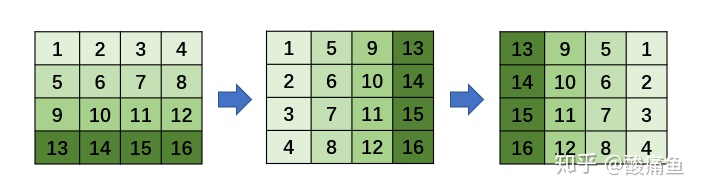因为这两种置换操作都是可逆的，所以通过这个方式，空间复杂为O(1)；而这种方式也是遍历两次matrix，遍历次数与第一种解法的一样。

def rotate(matrix):
n = len(matrix)

# 矩阵倒置，即(r,c)->(c,r)
for r in range(n):
for c in range(r, n):
matrix[r][c], matrix[c][r] = matrix[c][r], matrix[r][c]

# 每一行倒转
for r in range(n):
# 以下两行相当于：matrix[r] = matrix[r][::-1]
# 本着不修改matrix内部结构的原则，用下面的方式
for c in range(n // 2):
matrix[r][c], matrix[r][n - 1 - c] = matrix[r][n - 1 - c], matrix[r][c]

3

边整体旋转法

对于行列数都为n的矩阵，它总共有ceil(n / 2)圈（ceil表示向上取整）。我们可以通过对每一圈的四条边进行顺时针旋转来实现总体的效果。在边旋转过程中，我们需要先临时记录其中一条边的值，以便在最后将其放到目标的位置。所以边旋转法的空间空间复杂度为O(N)，而且只对matrix遍历了一次。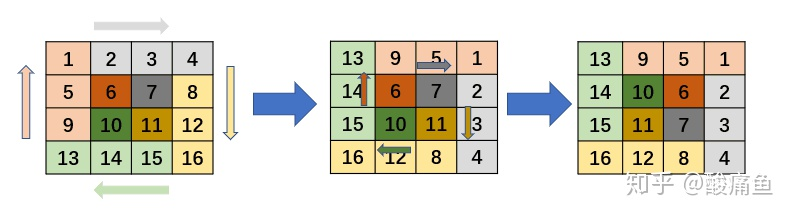每条边从起始位置旋转到目标位置的坐标转换公式，各位读者请自行推导，本文将不对此进行详细解说。

def rotate(matrix):
n = len(matrix)
mi = n - 1 # 最大索引

# 每一圈loop，对四条边单独旋转
for loop in range(n // 2):
# 每一圈边长
length = mi - 2 * loop

# 将左边存储到临时变量left中
left_c = loop
left_r_start = loop
left = [matrix[left_r_start + i][left_c] for i in range(length)]

# 将底边存到左边
bottom_c_start = loop
bottom_r = mi - loop
for i in range(length):
matrix[left_r_start + i][left_c] = matrix[bottom_r][bottom_c_start + i]

# 将右边存到底边
right_c = mi - loop
right_r_start = mi - loop
for i in range(length):
matrix[bottom_r][bottom_c_start + i] = matrix[right_r_start - i][right_c]

# 将上边存到右边
top_r = loop
top_c_start = mi - loop
for i in range(length):
matrix[right_r_start - i][right_c] = matrix[top_r][top_c_start - i]

# 将左边，即left存到上边
for i in range(length):
matrix[top_r][top_c_start - i] = left[i]

4

边元素依次旋转法

事实上，我们可以对每一圈的每条边上的每个元素单独进行旋转，这样的话，我们只需要临时记录其中的一个元素，以达到空间复杂度为O(1)的实现。

边元素旋转过程如图所示：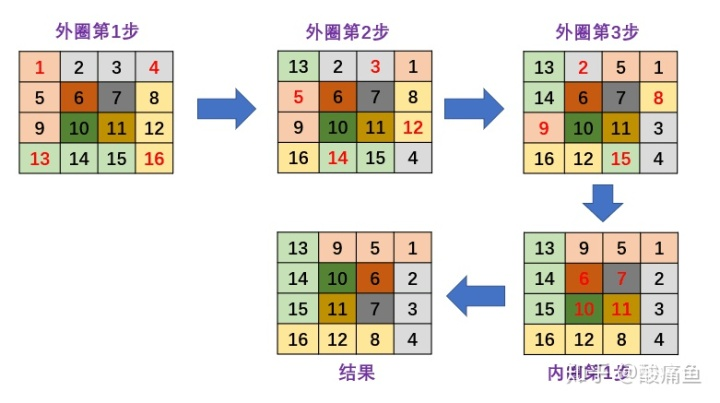def展开全文旋转矩阵算法
• 今天小编就为大家分享一篇python for循环输入一个矩阵的实例，具有很好的参考价值，希望对大家有所帮助。一起跟随小编过来看看吧
• Python= 46/365●有了数据就有了一切！回到枯燥而重要的数据部分。数据枯燥吗？一点儿都不。想想你看到的股票画面，想想你看到的成绩单，再想想你的信用卡账单?...创建一个新数组，并用结果填充。&g...
Python = 46/365

有了数据就有了一切！回到枯燥而重要的数据部分。数据枯燥吗？一点儿都不。想想你看到的股票画面，想想你看到的成绩单，再想想你的信用卡账单🤩🤩🤩哪里枯燥，一点儿也不……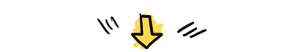日更不停，你看行不行？

我带着你，你带着Python Flag，共勉！

No.1 Python-NumPy数组操作(2)
(1)算术运算
数组上的算术运算符按元素应用。创建一个新数组，并用结果填充。
>>> a = np.array( [20,30,40,50] )>>> b = np.arange( 4 )>>> barray([0, 1, 2, 3])>>> c = a-b  >>> carray([20, 29, 38, 47])
以上是减法，表示对应元素的差值
#数组b的平方运算>>> b**2array([0, 1, 4, 9])
当然，当然，更复杂的来了！
>>> 10*np.sin(a)array([ 9.12945251, -9.88031624,  7.4511316 , -2.62374854])>>> a<35array([ True,  True, False, False])

(2)矩阵运算

与许多矩阵语言不同，乘积运算符*在NumPy数组中按元素进行运算。可以使用@运算符(在python> = 3.5中)或dot点函数或方法执行矩阵乘积：
>>> A = np.array( [[1,1],[0,1]] )>>> B = np.array( [[2,0],[3,4]] )>>> A * B array([[2, 0],       [0, 4]])>>> A @ Barray([[5, 4],       [3, 4]])>>> A.dot(B) array([[5, 4],       [3, 4]])
No.2 Python-NumPy数组索引、切片        一维数组可以被索引，切片和遍历，就像列表和其他Python序列一样。
>>> a = np.arange(10)**2>>> aarray([ 0,  1,  4,  9, 16, 25, 36, 49, 64, 81])>>> a4>>> a[2:5]array([ 4,  9, 16])

a [：6：2] = 1000;

从开始到位置6(不包括位置6)，将每个第二个元素设置为1000

>>> a[:6:2] = 1000>>> aarray([1000,    1, 1000,    9, 1000,   25,   36,   49,   64,   81])
No.3 Python-NumPy的Shape操作 shape 数组的形状 reshape改变数组的形状
>>> aarray([1000,    1, 1000,    9, 1000,   25,   36,   49,   64,   81])>>> a.shape(10,)>>> a.reshape(5,2)array([[1000,    1],       [1000,    9],       [1000,   25],       [  36,   49],       [  64,   81]])>>> a.shape(10,)
数组的形状可以使用各种命令来更改。请注意，以上命令均返回修改后的数组，但不更改原始数组。

一个人可以随意地阅读和学习，却不能随意地思考。

--叔本华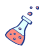• Python365专辑

Python，365！展开全文• 图二 ，剔除同一个目标上的重叠建议框，最终一个目标保留一个得分最高的建议框。图一图二2.那么如何实现非极大值抑制呢？步骤如下（假设进行非极大值抑制的输入为2000x20的矩阵，2000表示该图像上框的个数，20表示...

1.先解释什么叫IoU（intersection-over-union）。IoU表示(A∩B)/(A∪B) 即交并比。 非极大值抑制：图一 ——> 图二 ，剔除同一个目标上的重叠建议框，最终一个目标保留一个得分最高的建议框。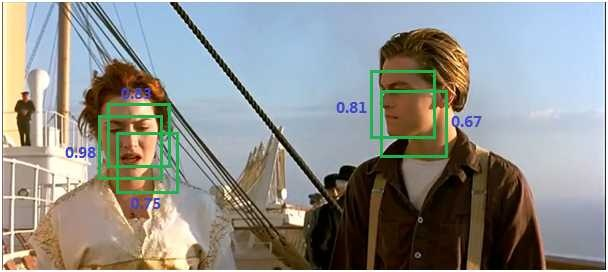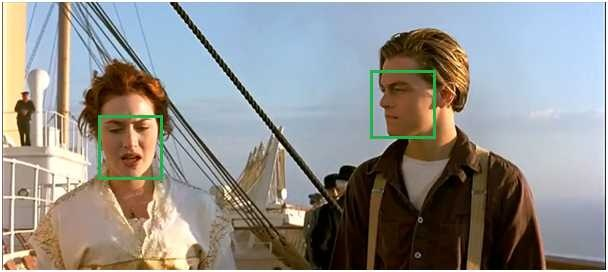2.那么如何实现非极大值抑制呢？步骤如下（假设进行非极大值抑制的输入为2000x20的矩阵，2000表示该图像上框的个数，20表示类别数）：

① 对2000×20维矩阵中每列按从大到小进行排序（每列表示一类，共20类。同一类可能有多个目标，如上图有两个人）；

② 从每列最大的得分建议框开始，分别与该列后面的得分建议框进行IoU计算，若IoU>阈值，则剔除得分较小的建议框，否则认为图像中同一类物体有多个目标；[两个同类的目标的建议框基本不会有重叠，因此去掉建议框重叠较大的实际上是实现了剔除同一个目标的重叠框]

③ 从每列次大的得分建议框开始，重复步骤②；

④ 重复步骤③直到遍历完该列所有建议框；

⑤ 遍历完2000×20维矩阵所有列，即所有物体种类都做一遍非极大值抑制；

3.代码实现如下，也可以在我的github上下载：https://github.com/hiJulie/NMS

import numpy as np
import random
import cv2

def non_max_suppress(predicts_dict, threshold):
for object_name, bbox in predicts_dict.items():#对每一个类别分别进行NMS；一次读取一对键值（即某个类别的所有框）
bbox_array = np.array(bbox, dtype=np.float)
#下面分别获取框的左上角坐标（x1，y1），右下角坐标（x2，y2）及此框的置信度；这里需要注意的是图像左上角可以看做坐标点（0,0），右下角可以看做坐标点（1,1），也就是说从左往右x值增大，从上往下y值增大
x1 = bbox_array[:, 0]
y1 = bbox_array[:, 1]
x2 = bbox_array[:, 2]
y2 = bbox_array[:, 3]
scores = bbox_array[:, 4]
order = scores.argsort()[::-1]#argsort函数返回的是数组值从小到大的索引值,[::-1]表示取反。即这里返回的是数组值从大到小的索引值
areas = (x2 - x1 + 1) * (y2 - y1 + 1)#当前类所有框的面积(python会自动使用广播机制，相当于MATLAB中的.*即两矩阵对应元素相乘)；x1=3,x2=5,习惯上计算x方向长度就是x=3、4、5这三个像素，即5-3+1=3，而不是5-3=2，所以需要加1
keep = []

#按confidence从高到低遍历bbx，移除所有与该矩形框的IoU值大于threshold的矩形框
while order.size > 0:
i = order
keep.append(i)#保留当前最大confidence对应的bbx索引
#获取所有与当前bbx的交集对应的左上角和右下角坐标，并计算IoU（注意这里是同时计算一个bbx与其他所有bbx的IoU）
xx1 = np.maximum(x1[i], x1[order[1:]])#最大置信度的左上角坐标分别与剩余所有的框的左上角坐标进行比较，分别保存较大值；因此这里的xx1的维数应该是当前类的框的个数减1
yy1 = np.maximum(y1[i], y1[order[1:]])
xx2 = np.minimum(x2[i], x2[order[1:]])
yy2 = np.minimum(y2[i], y2[order[1:]])
inter = np.maximum(0.0, xx2-xx1+1) * np.maximum(0.0, yy2-yy1+1)
iou = inter / (areas[i] + areas[order[1:]] - inter)#注意这里都是采用广播机制，同时计算了置信度最高的框与其余框的IoU
inds = np.where(iou <= threshold)#保留iou小于等于阙值的框的索引值
order = order[inds + 1]#将order中的第inds+1处的值重新赋值给order；即更新保留下来的索引，加1是因为因为没有计算与自身的IOU，所以索引相差１，需要加上
bbox = bbox_array[keep]
predicts_dict[object_name] = bbox.tolist()
#predicts_dict = predicts_dict
return predicts_dict

#下面在一张全黑图片上测试非极大值抑制的效果
img = np.zeros((600,600), np.uint8)
#predicts_dict = {'black1': [[83, 54, 165, 163, 0.8], [67, 48, 118, 132, 0.5], [91, 38, 192, 171, 0.6]]}
predicts_dict = {'black1': [[83, 54, 165, 163, 0.8], [67, 48, 118, 132, 0.5], [91, 38, 192, 171, 0.6]], 'black2': [[59, 120, 137, 368, 0.12], [54, 154, 148, 382, 0.13]] }
#在全黑的图像上画出设定的几个框
for object_name, bbox in predicts_dict.items():
for box in bbox:
x1, y1, x2, y2, score = box, box, box, box, box[-1]
y_text = int(random.uniform(y1, y2))# uniform()是不能直接访问的，需要导入 random 模块，然后通过 random 静态对象调用该方法。uniform() 方法将随机生成下一个实数，它在 [x, y) 范围内
cv2.rectangle(img, (x1, y1), (x2, y2), (255, 255, 255), 2)
cv2.putText(img, str(score), (x2 - 30, y_text), 2, 1, (255, 255, 0))
cv2.namedWindow("black1_roi")# 创建一个显示图像的窗口
cv2.imshow("black1_roi", img)# 在窗口中显示图像;注意这里的窗口名字如果不是刚刚创建的窗口的名字则会自动创建一个新的窗口并将图像显示在这个窗口
cv2.waitKey(0)# 如果不添这一句，在IDLE中执行窗口直接无响应。在命令行中执行的话，则是一闪而过。
cv2.destroyAllWindows()  # 最后释放窗口是个好习惯！

#在全黑图片上画出经过非极大值抑制后的框
img_cp = np.zeros((600,600), np.uint8)
predicts_dict_nms = non_max_suppress(predicts_dict, 0.1)
for object_name, bbox in predicts_dict_nms.items():
for box in bbox:
x1, y1, x2, y2, score = int(box), int(box), int(box), int(box), box[-1]
y_text = int(random.uniform(y1, y2))# uniform()是不能直接访问的，需要导入 random 模块，然后通过 random 静态对象调用该方法。uniform() 方法将随机生成下一个实数，它在 [x, y) 范围内
cv2.rectangle(img_cp, (x1, y1), (x2, y2), (255, 255, 255), 2)
cv2.putText(img_cp, str(score), (x2 - 30, y_text), 2, 1, (255, 255, 0))
cv2.namedWindow("black1_nms")# 创建一个显示图像的窗口
cv2.imshow("black1_nms", img_cp)# 在窗口中显示图像;注意这里的窗口名字如果不是刚刚创建的窗口的名字则会自动创建一个新的窗口并将图像显示在这个窗口
cv2.waitKey(0)# 如果不添这一句，在IDLE中执行窗口直接无响应。在命令行中执行的话，则是一闪而过。
cv2.destroyAllWindows()  # 最后释放窗口是个好习惯！

参考博客：

非极大值抑制(nms)算法功能及python实现 - CSDN博客

目标定位和检测系列（3）：交并比（IOU）和非极大值抑制（NMS）的python实现 - CSDN博

展开全文• 大家好，我是大师兄。很多同学抱怨自己很想学好Python，但学了好久，书也买不少，视频课程也看了不少，...今天我分享一些自己学习Python的心得，并用一个案例来说明python解决问题的基本思路和框架。1 如何学好Pytho...
• NumPy(Numerical Python)是Python种开源的数值计算扩展。这种工具可用来存储和处理大型矩阵，比Python自身的嵌套列表要高效的多，支持大量的维度数组与矩阵运算，此外也针对数组运算提供大量的数学函数库，在...
• Python中有一个非常大的二维数组，使用numpy库。我想要有效地遍历每一列，并且每次检查元素是否与0不同以在每列中计算它们的数量。在Python中高效地遍历numpy矩阵中的每一列假设我有以下矩阵。M = array([[1,2], [3,...
• 本文实例为大家分享了python实现二维数组的对角线遍历，供大家参考，具体内容如下 第种情况：从左上角出发，右下角结束 要完成的事情，就像下图： 话不多说，直接上Python实现代码与结果展示： # 输出遍历的索引...
• 在对SynthText的gt.mat中的wordBB中的数据进行处理时，我遇到一个问题：确保每一个值都在[0.,1.]内。如果用循环，就没有体现出python的逼格。于是找了三种方法(仅展示使每一个元素都小于1的方法)>>>import ...
• First, I tried to find an answer to my question ( which I think is... But this is no longer an issue since @ is possible (Python 3.5+) instead of nested dot calls. Therefore, by default, use numpy.array.
• 首先需要遍历二维数据，复习一下二维数组遍历的操作，操作时要注意数组每行的长度是否是一致的。 具体实例如下所示： # 要读取的nc数据是二维数组 EVEL_0h = EVEL[:][:] EVEL_3h = EVEL[:][:] EVEL_new ...
• 主要介绍了Python根据已知邻接矩阵绘制无向图操作,涉及Python使用networkx、matplotlib进行数值运算与图形绘制相关操作技巧,需要的朋友可以参考下
• 在定义一个修改数组元素的函数时，自己的代码修改不成功，不知道时什么原因，请教各位： 给一个数组的每个元素前面加上'The Great '几个字符 整体代码如下： def make_great(great_magicians): for great_magician ...有问必答
• 这两天写代码的时候遇到了，纪录一下，下次就不用去翻别人的了。 删除元素的时候呢，这样写： for i in a[:]: a.remove(i) 如果是修改元素，在遍历时如果想用迭代器对元素进行修改，是不会持久化的，这时候需要...列表
• 以上这篇Python遍历numpy数组的实例就是小编分享给大家的全部内容了，希望能给大家一个参考，也希望大家多多支持软件开发网。 您可能感兴趣的文章:numpy自动生成数组详解基于Python Numpy的数组array和矩阵matrix...
• 今天小编就为大家分享Python实现深度遍历和广度遍历的方法，具有很好的参考价值，希望对大家有所帮助。一起跟随小编过来看看吧
• 首先输入一个矩阵： >>> b=[[1,2,3,4,5,6],[2,2,3,4,5,6],[3,2,3,4,5,6],[4,2,3,4,5,6],[5,2,3,4,5,6]] >>> b=np.array(b) >>> b array([[1, 2, 3, 4, 5, 6], [2, 2, 3, 4, 5, 6], [3, 2, 3, 4, 5, 6], [4, 2, 3...
• 例如有一个包含个样本的训练集，你很可能习惯于用一个for循环来遍历训练集中的每个样本，但是当实现一个神经网络的时候，我们通常不直接使用for循环来遍历整个训练集，所以在这周的课程中你将学会如何处理训练集。...
• python for循环随机矩阵中的每一个元素 from numpy import*; #不用像as np 那样每次调用时输入np X=random.random((0,2)size(4,4))#创建一个只有0,1分布的4×4的随机矩阵 rows,cols=X.shape#读取X的行列数 for i in...随机矩阵
• 主要介绍了python实现树的深度优先遍历与广度优先遍历,详细分析了树的深度优先遍历与广度优先遍历原理及Python相关实现技巧,需要的朋友可以参考下
• new_list = ["H1", "H2", 1999, ["hello", "day", ["a", "b"]]] def loop_multi_array(list_string): for list_value in list_string: if isinstance(list_value, list): loop_multi_array(list_value) ...递归算法 多维数组
• 假设矩阵Matrix = [[1,2,3],4,5],[5,6,7]]分为3功能：def ContinSubSeq(lst):size=len(lst)for start in range(size):for end in range(start+1,size+1):yield (start,end)def getsubmat(mat,start_row,end_row,...
• python键值对数组 python录入学生成绩 全网python遍历二维数组搜索 python的二维列表的遍历 python构建二维数组 python二维函数遍历循环 python遍历数组的两种方法 python二维数组赋值 python遍历array python二维...
• Python数据挖掘基础Numpy如何优雅的遍历一个数组，使用嵌套for循环吗？当我们想要遍历一个多维数组的时候，如果通过不停的使用循环去遍历，这会让我们的代码可读性与性能都变得很差，numpy提供了一个函数nditer,以...
• 1. 引言 Numpy是Python中常见的数据处理库。Numpy是 Numerical Python的缩写，...首先我们来看例子，使用循环来遍历数组，样例代码如下： import numpy as np array = np.array([1, 2, 3, 4, 5, 6]) for x in array:编程技巧
• I am trying to study the probability of having a zero value in my data and I have developed a code that outputs the value of a column of data when the other is zero which is what I need.......